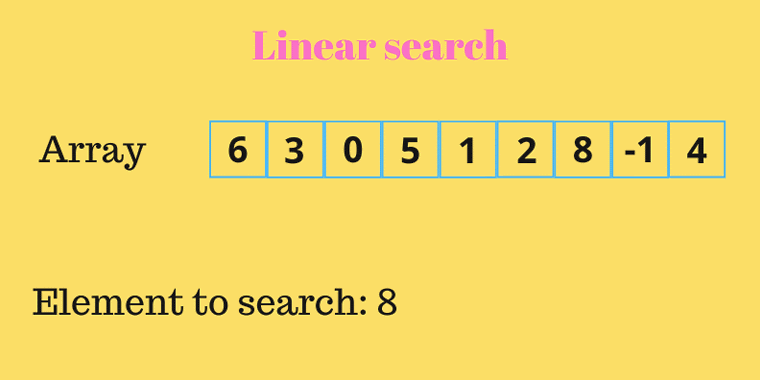#### 2 时间复杂度

O(n)，意味着随着数组元素越来越多，则查找的时间将越来越长。即查找时间与数据规模成正比。注意：时间复杂度通常是指最差的时间复杂度，而不能单独去最优的时间复杂度。如：有1，2，3，，，100000的数组，通过线性查找的方式，从中找数据1，立即就能找到。但是，不能说，此时的线性查找的时间复杂度是O(1)。

#### 4 代码实现

```public class LinearSearch{
public int search(int[] arr,int key){
for(int i=0;i<arr.lenth;i++){
if(arr[i]==key)
return i;
}
return -1;
}
public static void main(String[] args){
int[] arr={6,2,4,3,1,5};
int key=1;
LinearSearch ls=new LinearSearch();
int index=ls.search(arr,key);
System.out.println("index of "+key+" is: "+index);
}
}```

#### 5 代码改良

```public class LinearSearch{
//私有化构造方法，防止外面通过实例化本类的对象来调用search()方法
private LinearSearch(){}

//为了实现线性查找可以支持任意类型的函数，这里只需要将该方法改为泛型方法即可，而无需将该类改为泛型类
//另外，改为泛型方法之后，就要通过equals()来判断是否相等，而不能再使用==比较对象是否相等了，因为==比较的是对象地址是否相等，而equals()比较的才是内容。
public static <T> int search(T[] arr,T key){
for(int i=0;i<arr.length;i++){
if(arr[i].equals(key)){
return i;
}
}
return -1;
}

public static void main(String[] args){
//由于方法是泛型方法，这里就必须要用类类型的数组来调用，而不能使用基本数据类型的数组
Integer[] arr={6,2,4,3,1,5};
int key=1;
int index=LinearSearch.search(arr,key);
System.out.println("index of "+key+" is: "+index);
}
}```

#### 6 代码扩展

```  public class User {
private String name;
private int age;

public User(String name, int age) {
this.name = name;
this.age = age;
}

@Override
public boolean equals(Object o) {
if (this == o) return true;
if (o == null || getClass() != o.getClass()) return false;

User user = (User) o;

if (age != user.age) return false;
return name != null ? name.equals(user.name) : user.name == null;
}
}```

```        User u1 = new User("Jim", 10);
User u2 = new User("Kate", 8);
User u3 = new User("Lilei", 12);
User[] users = {u1, u2, u3};
int zz = LinearSearch.search(users, new User("Kate", 8));
System.out.println(zz);```

#### 7 性能测试

```public class ArrayGenerator{
//构造方法同样设置为private
private ArrayGenerator(){}
//接收1个整数n,构建1个长度为n的Integer[],并且元素值分别为0,1,2,...n-1
public static Integer[] generateOrderedArray(int n){
Integer[] arr=new Integer[n];
for(int i=0;i<arr.length;i++){
arr[i]=i;
}
return arr;
}
}```

```public class LinearSearch{
//构造方法私有化，避免在类外部实例化该类的对象
private LinearSearch(){}
//公共的静态的支持泛型的线性查找法
//传入一个T类型的数组arr和T类型的元素key，线性遍历该T数组，如果发现有元素和key值相等，则返回该元素在数组中的下标，意味着已经找到，结束查找。如果遍历完整个数组，都找不到和key值相等的元素，则意味着该数组中不包含该元素key，返回-1意味着查找失败。
public static <T> int search(T[] arr,T key){
for(int i=0;i<arr.length;i++){
if(arr[i].equals(key)){
return i;
}
}
return -1;
}

public static void main(String[] args){
//预定义1个数组，元素分别为10万，100万
int[] dataSize = {100000,1000000};
//foreach循环，相当于分别把10万，100万作为参数，用于创建长度也为10万、100万的线性有序数组
for(int n:dataSize){
Integer[] arr = ArrayGenerator.generateOrderedArray(n);
long beginTime = System.currentTimeMillis();
//调用线性查找法去遍历长度为10万、100万的数组，从中查找元素也为10万、100万的元素
LinearSearch.search(arr,n);
long endTime = System.currentTimeMillis();
System.out.println("Array size: "+n+" key: "+n+" Time: "+(endTime-beginTime));
}

//支持自定义类User的线性查找
User u1 = new User("Jim", 10);
User u2 = new User("Kate", 8);
User u3 = new User("Lilei", 12);
User[] users = {u1, u2, u3};
int zz = LinearSearch.search(users, new User("Kate", 8));
System.out.println(zz);
}
}```